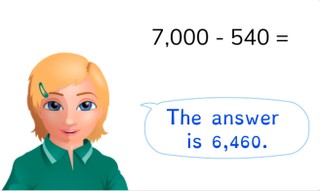Subtraction to 10,000 with simple numbers

# Subtraction to 10,000 with simple numbers

Students learn to subtract to 10,000 with simple numbers.8,000 schools use Gynzy92,000 teachers use Gynzy1,600,000 students use Gynzy

## General

Students learn subtraction to 10,000 with simple numbers.

## Standards

CCSS.Math.Content.4.NBT.B.4

## Learning objective

Students will be able to subtract to 10,000 with simple numbers.

## Introduction

Have the students calculate how many passengers eventually arrive in America. Have them calculate out loud how many passengers there are in the airplane after each stop.

## Instruction

Explain that there are different ways in which you can subtract to 10,000 with simple numbers. Show the example of the girl, who solves the problem by simplifying the numbers. Then show the example of the boy who solves the problem by splitting the numbers. Have the students solve the following two problems on their own. Afterwards, discuss the different methods of solving the problem. Now show the problem 7,800 - 531. To solve this problem, the girl makes the number one less. The other girl solves the problem by splitting up the number. Have the students solve the next two problems on their own. Then discuss the different methods to solve the problem. For the story problem, you start by taking the problem out of the story. Then you show which methods you can use to solve the problem in steps. Have the students solve the next two story problems on their own.

Check whether the students can subtract to 10,000 with simple numbers using the following question:
- How can you subtract to 10,000 with simple numbers in steps?

## Quiz

The students are given ten exercises in which they practice subtraction to 10,000 with simple numbers. Some problems are given a visual aid, others are in the abstract, and the rest are in story form.

## Closing

Discuss with the students the importance of subtracting to 10,000 with simple numbers. As a closing activity you can have the students listen to the starting number and then the following speaker, to hear how much they must subtract from the starting number. The final answer can be checked at the end of each route.

## Teaching tips

Students that have difficulty with subtracting to 10,000 with simple numbers can first practice by subtracting to 1,000. Start with subtraction of a ones value (for example 1,000 - 3), then a tens number, then a hundreds number and then a combination of ones values, tens numbers and hundreds numbers. Use MAB blocks to show the problem visually.

## Instruction materials

MAB blocks

### The online teaching platform for interactive whiteboards and displays in schools

• Save time building lessons

• Manage the classroom more efficiently

• Increase student engagement